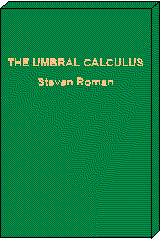##The Umbral Calculus

#### Pure and Applied Mathematics, Volume 111

This monograph is intended to be an elementary introduction to the modern umbral calculus. Since we have in mind the largest possible audience, the only prerequisite is an acquaintance with the basic notions of algebra and perhaps a dose of applied mathematics (such as differential equations) to help put the theory in some mathematical perspective.

### Contents

• Chapter 1 - Introduction
• Chapter 2 - Sheffer Sequences
• Chapter 3 - Operators and Their Adjoints
• Chapter 4 - Examples
• Chapter 5 - Topics
• Chapter 6 - Nonclassical Umbral Calculi

1984, ISBN 0-12-594380-6, 193 pp., Hardcover